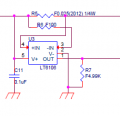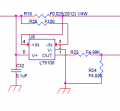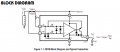#### Chandler Timm Doloriel

Joined Apr 5, 2018
52
Hi folks,

I'm doing a project that involves reading a current from a supply line. To read the current using PIC16F1789, I used its ADC function (8-bit @ 5V) and LTC6106 for current sensing. However, I don't know how to convert the ADC reading to actual current sensed. I followed the formula and schematic in the LTC6106 datasheet but still not getting the correct results.

This is the 1st configuration: 3v3 Supplyof which I read HEX:16 and the expected reading should be 173mA.

This is the 2nd configuration: 5v0 Supplyof which I read HEX:07 and the expected reading should be 55mA.

I followed this formula and schematic in the datasheet but still no luck.For someone that can help, I am very thankful that you shared your knowledge.

Regards,
Chandler

Last edited:

#### KeithWalker

Joined Jul 10, 2017
1,301
What range of current do you want to measure? The resolution will be about 7mA with the current setup. Is that good enough? Is it just a matter of applying a correction factor to the results or do you have other problems?

#### Chandler Timm Doloriel

Joined Apr 5, 2018
52
What range of current do you want to measure? The resolution will be about 7mA with the current setup. Is that good enough? Is it just a matter of applying a correction factor to the results or do you have other problems?
What is the formula to convert HEX:16 and HEX:07 into current reading?

#### ronsimpson

Joined Oct 7, 2019
1,020
What is the formula to convert HEX:16 and HEX:07 into current reading?
You need to know if you are using the ADC in 8 bit mode or 12 bit mode.
What is the reference voltage for the ADC? There is too many choices. Depending on which micro, supply is one option, external voltage, or 1/2 supply, or 1.25V or .....

If Vref=5.0V supply and 8 bit mode then each bit is 5V/256= 0.0195 volts.
0=0, 1=0.0195, 2=0.039, 3=0.05859 .... at 2.5V you should read 7Fh or 80h .... at 5V = FFh.

#### Chandler Timm Doloriel

Joined Apr 5, 2018
52
You need to know if you are using the ADC in 8 bit mode or 12 bit mode.
What is the reference voltage for the ADC? There is too many choices. Depending on which micro, supply is one option, external voltage, or 1/2 supply, or 1.25V or .....

If Vref=5.0V supply and 8 bit mode then each bit is 5V/256= 0.0195 volts.
0=0, 1=0.0195, 2=0.039, 3=0.05859 .... at 2.5V you should read 7Fh or 80h .... at 5V = FFh.

#### ronsimpson

Joined Oct 7, 2019
1,020
Read the data sheet for the IC. It turns current into voltage.

#### KeithWalker

Joined Jul 10, 2017
1,301
This is a link to a conversion utility:
https://www.rapidtables.com/convert/number/hex-to-decimal.html#:~:text=Hex to decimal conversion table , 3 46 more rows
Hex 07 = Dec 7
Hex 16 = Dec 22
The full range of your 8 bit D/A is Dec 0 to 255
Both of these readings are very small values for the range of the A/D so a conversion factor from them will have a large error margin.
Resolution per D/A bit = current/reading = 173/22 = 7.86 mA
You will get a much more accurate conversion result if you measure a current value much closer to full scale which is about 2A.
Regards,
Keith